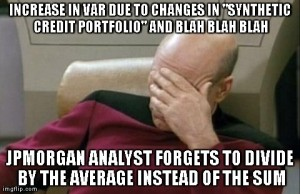# Modeloff 2013 Excel Challenge #3 Follow Up Explanation

Sometimes when an Excel expert provides you with advice, it makes sense to write it down somewhere and share it with people you know will find this advice useful. This is exactly what this post is about. As a follow up to Challenge #3 we posted last week, Dan Mayoh (the creator of the challenge) provided us with words of wisdom on how to apply the lessons from this challenge to everyday financial modeling. The response below was edited and we added in our own comments where necessary. You can reach Dan at dan@fintega.com.## The Truth Behind TRUE/FALSE Arrays

We mentioned the step where we needed to convert the TRUE/FALSE array into numbers in order to sum them. We were spot on with why it works when you do SUM((E20:S59>10)+(E20:S59<-10)), but not with =SUM(ABS(E20:S59)>10).

When Excel evaluates equalities and inequalities (expressions using the ‘=’, ‘<’ or ‘>’ operators for example), that little part of the equation evaluates to TRUE or FALSE. Typically we then want Excel to then resolve the TRUE/FALSE values into 1/0 values. The SUM() function alone won’t do it, but the ‘+’ operator will.

This is actually a really common occurrence in financial modelling. In this example, we needed to resolve the TRUE/FALSE values into 1/0 values so we could then sum them. More common in financial modelling might be a situation where we are modelling a row of indicators or flags, such as “is the current period before the operations start date (in which case return 0) or after the operations start date (in which case return 1)?”. Additionally, the model will look neater if it displays the results as “1” and “0” rather than “TRUE” and “FALSE”.

## Downsides of Using the IF() Function

If the formula was written as =IF(ColumnPeriodEndDate > OpsStartDate, 1 , 0) then there is no issue. But a savvy financial modeler will not use an IF() function here. Since only results of 1 or 0 returned, which are equivalent to TRUE and FALSE, the IF() function is a bit redundant. Much better (and quicker from a computational point of view) is to simply convert the TRUE/FALSE results into 1/0. Hence they will base the formula around the inequality =(ColumnPeriodEndDate > OpsStartDate), and then convert the Boolean result into a number.

## Converting a Boolean Result to a Number

There are a few ways to do this. The most common is to perform a mathematical operation, such as ‘+’ or ‘*’. Specifically, it is common to either:

1. Include “1*” in front or “*1” at the end of the expression. Multiplying the TRUE/FALSE value by 1 which converts it into 1 or 0.
2. Include “–” in front of the expression. That is, “minus minus” or two negative signs. This essentially performs multiplication by negative 1 twice, the same as multiplying by 1. And indeed this is why in financial models you’ll sometimes see formulas starting with “minus minus”.

Doing the “*1” in front of a Boolean expression is something we totally forgot about! The “minus minus” tip is something we have never encountered in our models, but this is definitely a simple solution to quickly convert the Boolean to a number. – KeyCuts Team Comment

## Do People Actually Use the N() Function?

As we discovered from doing a quick Google search, another way to achieve the same result is using the N() function, and it is 1 character shorter than “1*” or “–”! But in real world practice, it’s not common to use the N() function here.

The N() function IS sometimes used in real world financial models. It can be a useful way of including an annotation or comment WITHIN a formula to help describe what the formula is doing. If the formula is a bit unusual or complicated, N() allows the author to provide some guidance. You simply include at the end of the formula the expression +N(“your choice of explanatory text”). The N() function will convert any text value to 0, meaning that we are simply adding 0 on to the end of the formula, which won’t change the result. But it allows us to leave a written record within the formula.

This is genius! Instead of leaving the typical yellow sticky comment, this is an in-line method to write comments within your model. – KeyCuts Team Comment

## Conclusion

Understanding the nuances of why an Excel formula works is interesting and gets you thinking about optimization, but the real world application is all that really matters when your job is on the line. Excel can be all fun and games but not thinking about how your colleagues may use your Excel file or keeping the file size down in the event of large amounts of data crunching may lead to disaster. Even worse, you get caught up with writing the best formula and huge mistakes like the JPMorgan VaR error can occur.